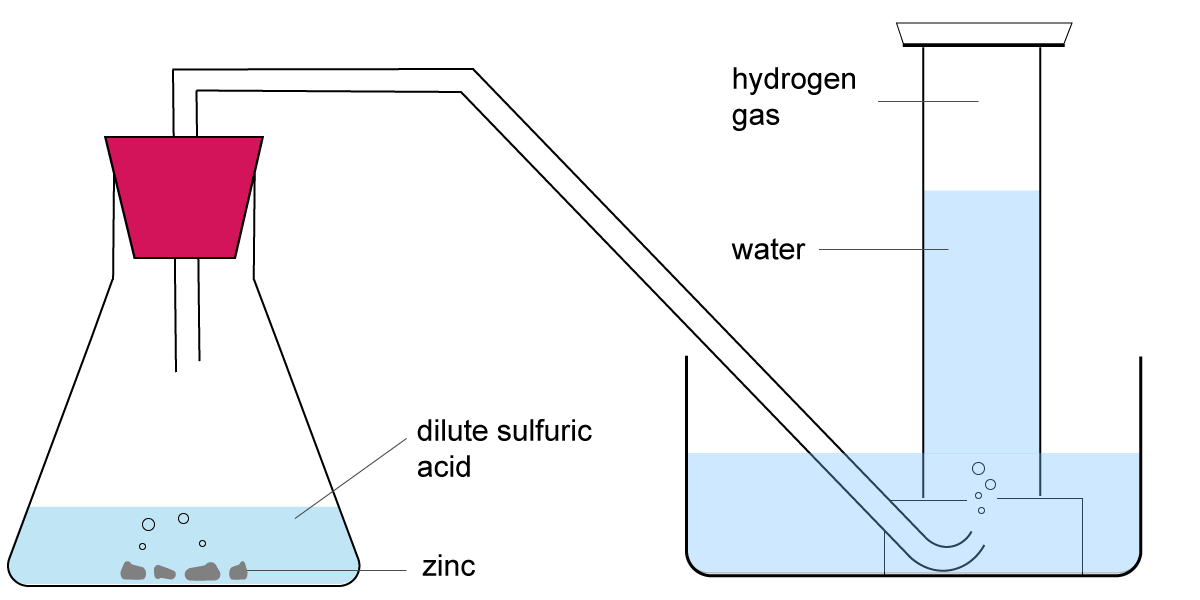Chemistry Edexcel GCSE:
Extracting Metals

Page 1

You will need a copy of the periodic table!

1. Four metals, W, X, Y and Z, were added separately to water and to dilute hydrochloric acid. The observations are given in the table below:

 Metal Reaction with water Reaction with acid W No reaction No reaction X Bubbles slowly Bubbles vigorously Y No reaction Bubbles slowly Z Bubbles slowly Bubbles slowly

What is the order of reactivity of these metals from most to least reactive?

• A.   X , Z , W , Y
• B.   X , Z , Y , W
• C.   X , Y , Z , W
• D.   W , Y , Z , X

2. Metals S, T and U were extracted from their ores by the following methods:

 Metal Extraction Method S Occurs naturally as the metal T Electrolysis of molten oxide U Heating metal oxide with carbon

What is the order of reactivity of these metals from most to least reactive?
• A.   S > U > T
• B.   T > S > U
• C.   T > U > S
• D.   U > T > S

Q3-4:
The reactivity of three different metals, P, Q and R, were investigated by reacting the metals with each other’s sulfate solutions.
The results are given in the table below. A tick indicates a reaction and a cross indicates no reaction.

 Metal P Metal Q Metal R Sulfate of P x x ✔ Sulfate of Q ✔ x ✔ Sulfate of R x x x

3. What is the order of reactivity of these metals from most to least reactive?
• A.  R, P, Q
• B.  Q, P, R
• C.  P, Q, R
• D.  R, Q, P
4. The metal sodium cannot be used in this investigation.The reason that sodium cannot be used is..
• A.   sodium is stored under oil
• B.   sodium does not form a sulfate salt
• C.   sodium will react vigorously with the water in the solution
• D.   sodium is too expensive
5. Which statement is true?
• A.   The more reactive a metal the easier it is to reduce the metal
• B.   The more reactive a metal the easier it to oxidise the metal
• C.   The less reactive a metal the easier it is to reduce the metal
• D.   The less reactive a metal the easier it is to oxidise the metal
6.Why does aluminium need to be extracted from its ore using electrolysis rather than heating with carbon?
• A.   Electrolysis is a cheaper process than heating with carbon
• B.   Aluminium is more abundant than iron in the Earth’s crust
• C.   Aluminium is an expensive metal
• D.   Aluminium is more reactive than carbon

Q7-8:
A strip of copper metal was placed in a boiling tube containing silver nitrate solution and left to react. The equation for this reaction is:

Cu(s)  + 2AgNO3(aq)  Cu(NO3)2(aq) + 2Ag(s)

7. In this reaction explain whether the copper is oxidized or reduced.

 Copper is.. Because copper.. A oxidised Lost electrons B oxidised Gained electrons C reduced Lost electrons D reduced Gained electrons
8. This reactions shows us that..
• A.   copper is more reactive than silver
• B.   silver is more reactive than copper
• C.   copper is more reactive than silver nitrate
• D.   silver nitrate is more reactive than copper

9. The following statements relate to the extraction of metals.

 I. Unreactive metals are often found as uncombined elements II. Aluminium is more difficult to extract than copper III. Iron is extracted from its ore by heating with carbon

Which of these statements are true?

• A.   I and II only
• B.   I and III only
• C.   II and III only
• D.   I, II and III
10. A student used zinc and dilute sulfuric acid in the following experiment to collect a sample of hydrogen gas:Which two other metals could the student have safely used to produce a sample of hydrogen?

• A.   copper and silver
• B.   potassium and magnesium
• C.   lithium and copper
• D.   magnesium and iron